# Translations of Encyclopedia about Mathematics

## Proportion

On some models of cars, we can find the following label for children: scale of 1:100 to the original. This proportion is exactly what we will be discussing now.

So what does a scale of 1:100 mean exactly?

From a mathematical point of view, we have two values here: the size of the model (h) and the size of the original (o), which gives us a proportion of 1 to 100. Therefore: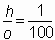The proportion of the model is therefore 0.01 of the original. A proportion is calculated by dividing two know values. In our case, that was 1 : 100 = 0.01.

Direct Proportion

If two dependent values change (by multiplication or subtraction) and the coefficient expressing their proportion remains the same, we call it a direct proportion. The proportion of two values is expressed as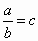. If c is a constant, then the proportion is direct.

Example:

A kilo of fish costs 25 DM, in which case its coefficient is directly proportional: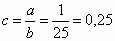.

If some of the values change and the direct proportion remains the same, then the other value must increase proportionally.

Indirect Proportion

If, with a constant coefficient of proportion, one of the values increases and the other decreases, we have an indirect proportion. This means that if one of the values doubles in size, the other must decrease in size by half. The relation between these two values can be expressed by a coefficient of indirect proportion c = a . b, which remains constant.

Example:

If two workers need 5 hours to load three trucks, how many hours would 6 workers need to do the same job? In this case, the coefficient of indirect proportion is c = a . b = 2 . 5 = 10.

If the value of a increases three fold (we have 6 workers) then, while keeping the value of c constant, b would have to become one third its size.

6 · b = 10 | :6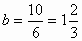Test: 5 : 3 =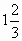Translating Dutch Hungarian Translations Hungarian Dutch Translating Swedish Czech Translations Czech Swedish Translating RussianEnter your search terms Submit search form